# 一、强化学习相关背景知识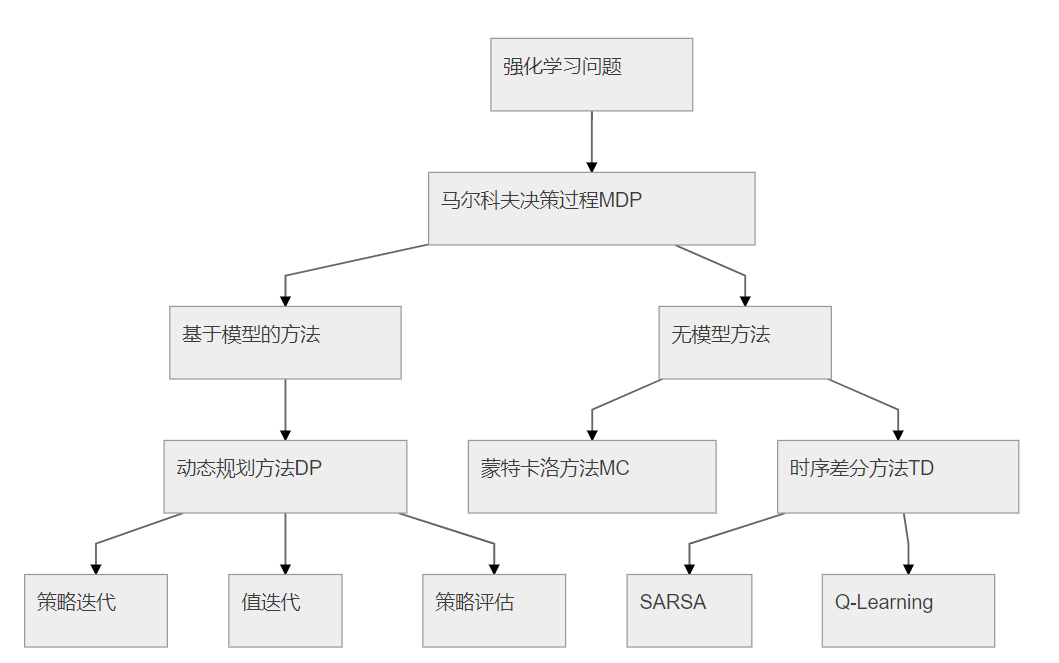# 二、Neural Architecture Search with Reinforcement Learning

## 1、总览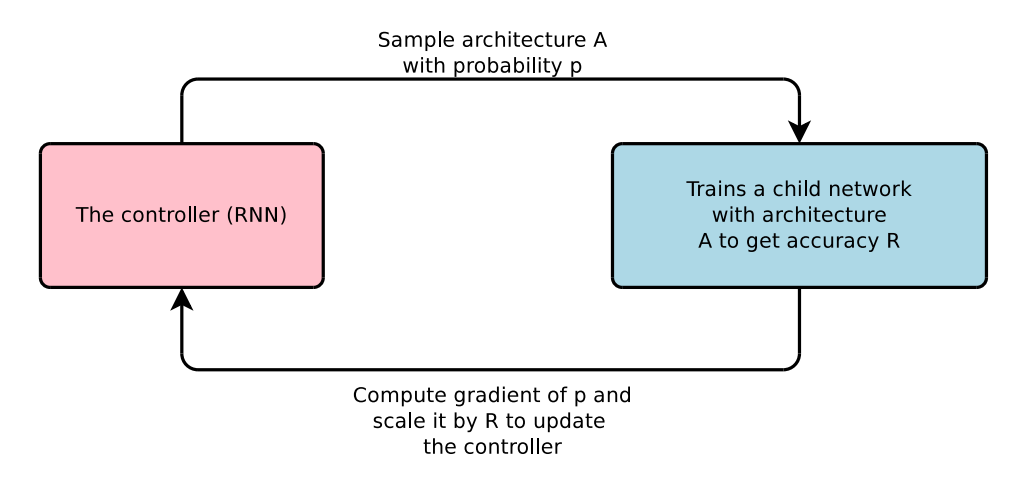## 2、控制器RNN的实现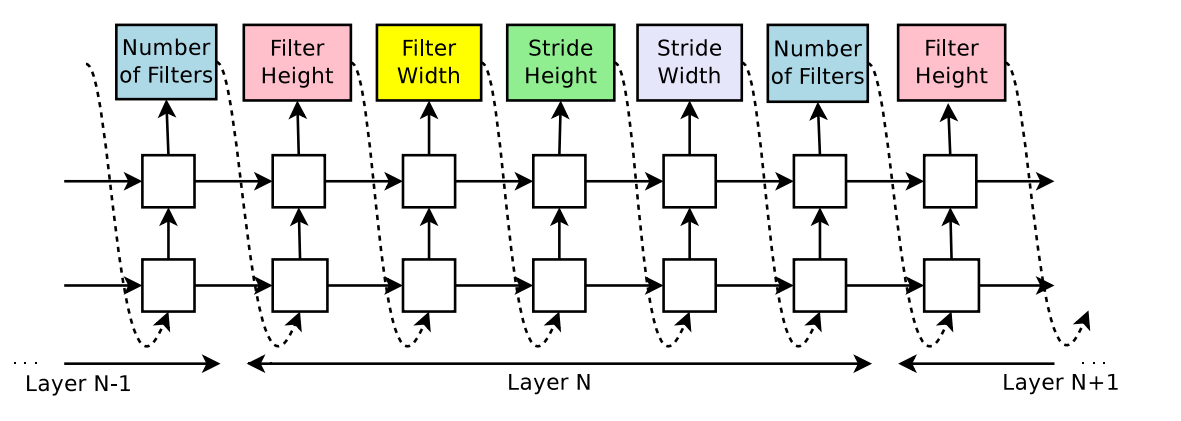RNN会预测每一层的滤波器的高度、宽度，步长的高度、宽度，和滤波器个数，一个5个参数。每一个预测是通过softmax分类器实现的，这个时间步的预测会作为下个时间步的输入。当层数达到一个特定的值，这个生成的过程就会停止。这个值会随着训练过程增加。一旦RNN生成了一个结构，即子网络，这个子网络就会被训练，验证集的准确率被记录下来被作为奖励。然后控制器RNN的参数$\theta _{c}$被更新以生成期望验证集准确率最大的结构。

## 3、策略梯度

RNN生成一个子网络后，其生成的标记序列可以被看作一系列动作$a_{1:T}$。验证集在其上取得的准确率作为奖励$R$，然后训练RNN。为了找到最优的结构，我们网RNN取最大化期望奖励$J(\theta _{c})$：

## 4、增加网络复杂度：跨连接和其他类型的层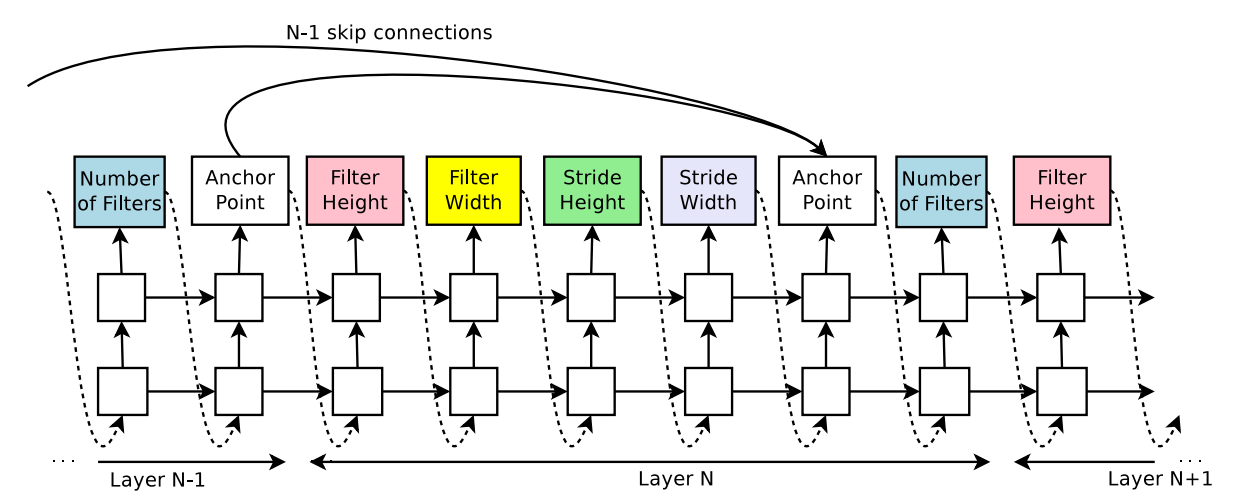$h_{j}$是RNN在第$j$层锚点的隐藏状态，$j$从0到N-1。然后从这些sigmoid中参与决定当前层和前几层中的哪些连接。矩阵$W_{prev}, W_{curr}, v$是可训练的参数。

## 5、生成循环神经网络的cell

RNN和LSTM的cell可以看做以$h_{t-1}, x_{t}$为输入去生成$h_{t}$的一系列步骤的树状结构。控制器需要标记树种每个节点的连接方式（加、对应元素相乘等）和激活函数（tanh，sigmoid等），然后融合两个输入作为输出。然后两个输出再作为下个节点的输入。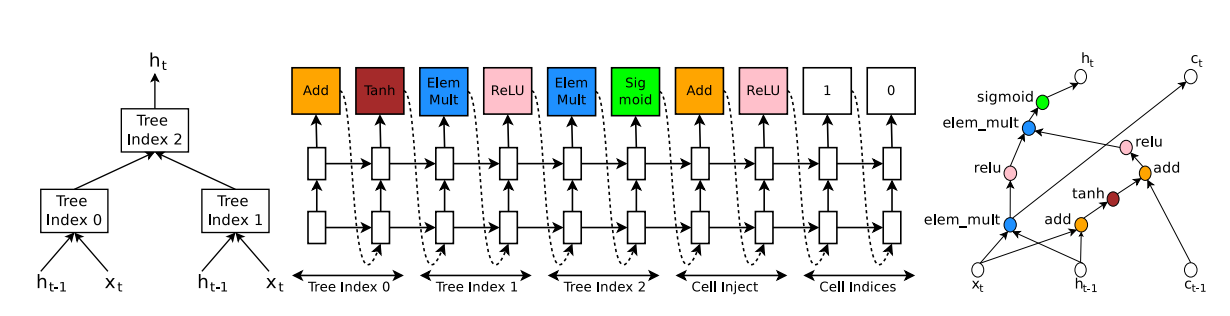• 对于节点0，控制器预测了Add和Tanh，即：$a_{0}={\rm tanh} (W_{1} \ast x_{t}+W_{2} \ast h_{t-1})$
• 对于节点1，控制器预测了ElemMult​和​ReLu​，即：$a_{1}={\rm ReLU}((W_{3} \ast x_{t}) \odot (W_{4} \ast h_{t-1}))$
• 在cell index这个block里，第二个元素预测了0，表示$c_{t-1}$会连接节点0；cell inject这个block里，预测了Add和ReLu，即节点0的输出会和$c_{t-1}$重新连接：$a_{0}^{new}={\rm ReLu}(a_{0}+c_{t-1})$
• 对于节点2，控制器预测了ElemMult​和sigmoid，即$h_{t}=a{2}={\rm sigmoid}(a_{0}^{new}\bigodot a_{1})$
• 在cell index这个block里，第一个元素预测了1，表示将节点1未经激活函数的输出作为$c_{t}$，即：$c_{t}=(W_{3} \ast x_{t}) \odot(W_{4} \ast h_{t-1})$

# 三、Designing Neural Network Architectures Using Reinforcement Learning

## 2、搜索方法总览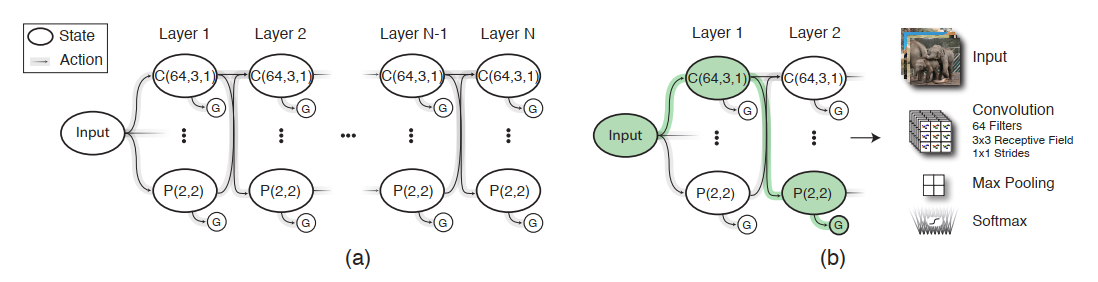## 3、状态空间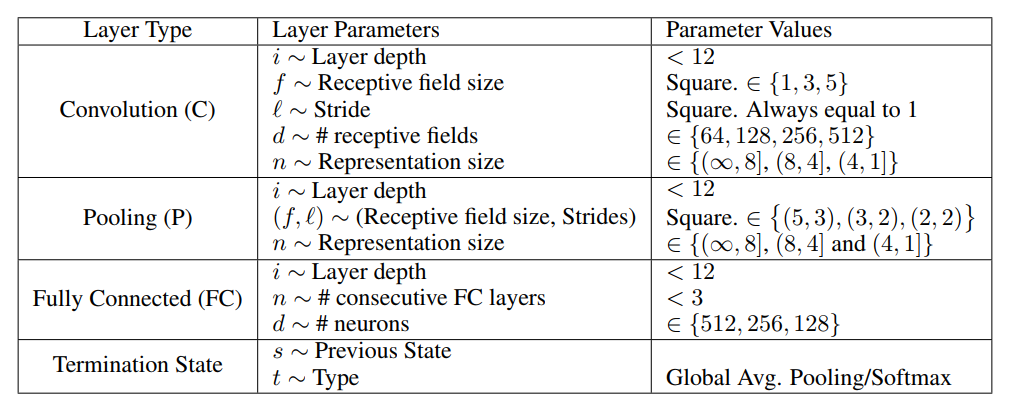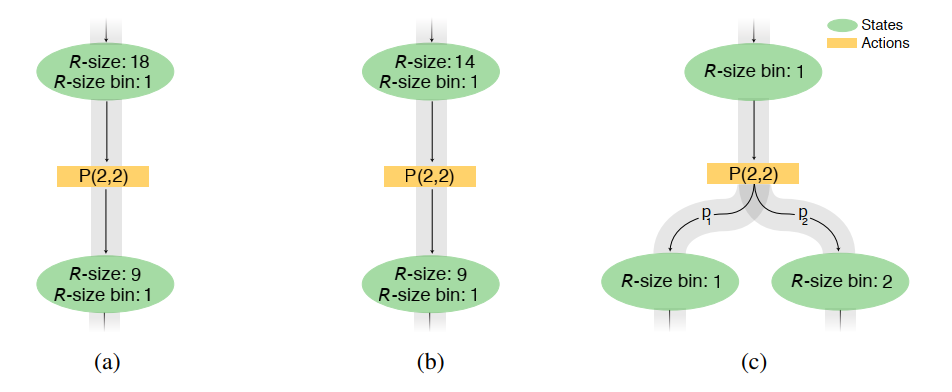## 5、Q-learning训练过程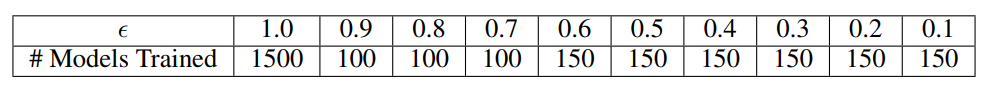## 6、伪代码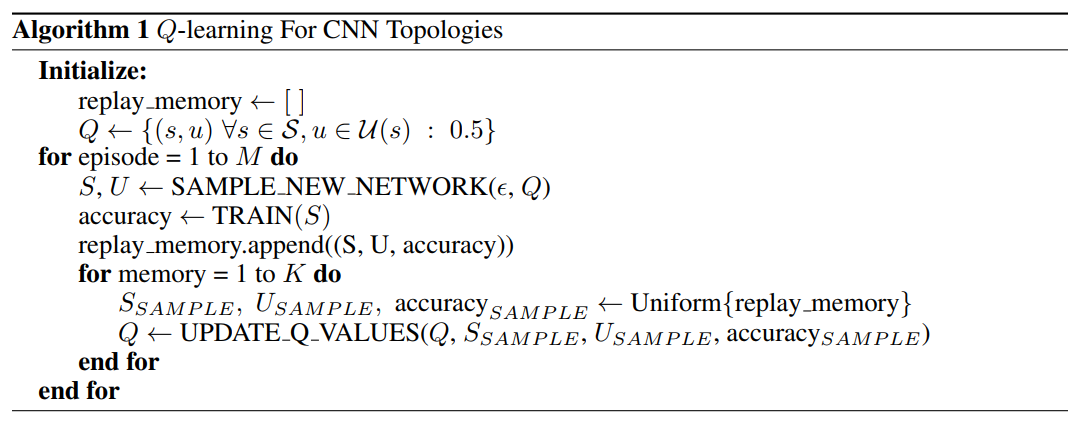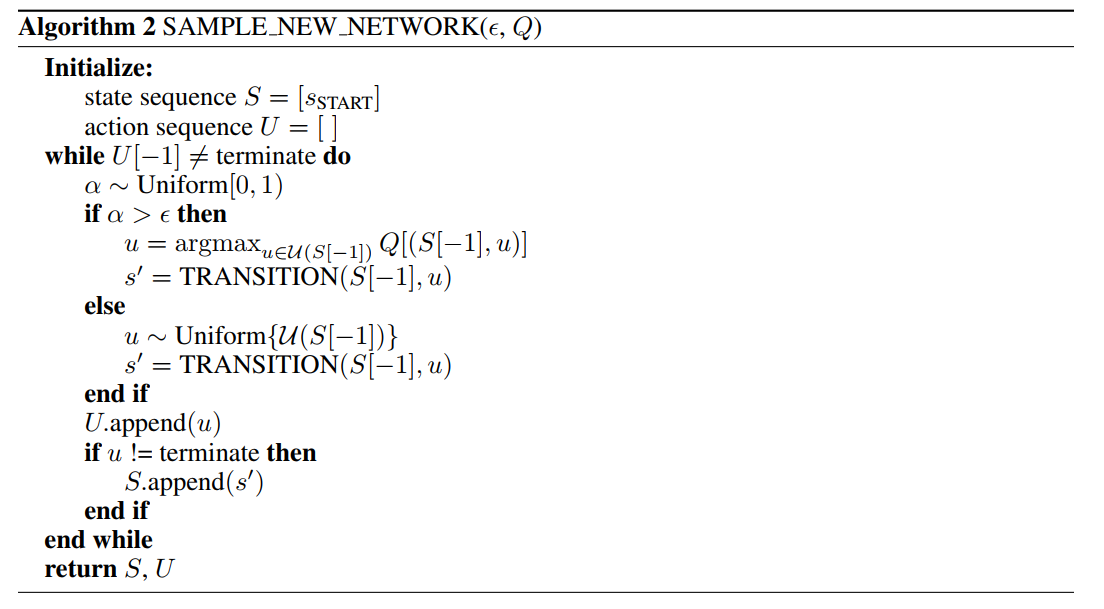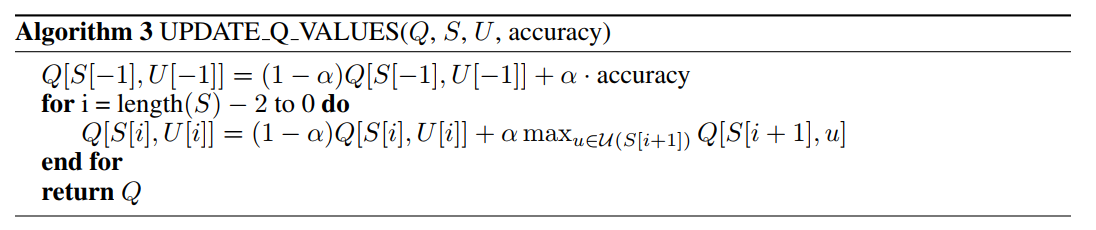# 参考文献

1. https://www.automl.org/best-practices-for-scientific-research-on-neural-architecture-search/
2. Zoph B , Le Q V . Neural Architecture Search with Reinforcement Learning[J]. 2016.
3. Designing Neural Network Architectures using Reinforcement Learning
4. https://blog.csdn.net/u014380165/article/details/78525500
5. https://blog.csdn.net/xjz18298268521/article/details/79078835/
6. 《深入理解AutoML和AutoDL》